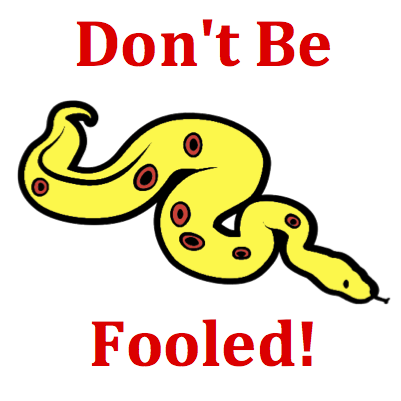# Kinematic Graphing - Mission KG7 Detailed HelpThe motion of six objects - A, B, C, D, E, and F - are represented by lines on a velocity-time graph. Write the letter(s) for any object that changes its direction. List all that apply ... .Velocity-Time Graphs: Velocity versus time graphs represent changes that occur in an object's velocity with respect to time. The slope of the line is the acceleration (change in velocity divided by the change in time) of the object. The area under the line (between the line on the graph and the time axis) is the displacement of the object.This question asks you to identify any object that changes its direction. Many students confuse this question with another question. They mistranslate the question into "Write the letter of any line which changes its direction." Don't be fooled! These are two different questions. A plotted line on a velocity graph could change its direction (that is, be sloping up and then later be a horizontal line) without the object itself changing direction.This is an example of a question in which you have to use your noodle(that's your brain). Velocity is a vector with a magnitude and a direction. The direction that an object is moving is often represented by a + or - sign on the velocity. An object with a negative velocity may be moving leftward or downward since these directions are often assumed to be the negative direction. The velocity-time graph displays a plot of velocity values - either in the positive region of the graph (above the time axis) or in the negative region (below the time axis). If an object is changing the direction it is moving, then it is changing its velocity value from a positive value to a negative value. The graph of such a motion would be represented by a line that crosses over the axis from the positive region to the negative region (or vice versa).